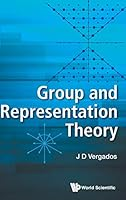# Group and Representation Theory## Book Description

This volume goes beyond the understanding of symmetries and exploits them in the study of the of both classical and . Thus it is important to study the symmetries described by continuous (Lie) groups of transformations. We then discuss how we get operators that form a Lie algebra. Of particular interest to physics is the representation of the of the algebra and the group in terms of matrices and, in particular, the irreducible representations. These representations can be identified with physical observables.This leads to the study of the classical Lie algebras, associated with unitary, unimodular, orthogonal and symplectic transformations. We also discuss some special algebras in some detail. The discussion proceeds along the lines of the Cartan-Weyl theory via the root vectors and root diagrams and, in particular, the Dynkin representation of the roots. Thus the representations are expressed in terms of weights, which are generated by the application of the of the algebra on uniquely specified highest weight states. Alternatively these representations can be described in terms of tensors labeled by the Young tableaux associated with the discrete symmetry Sn. The connection between the Young tableaux and the Dynkin weights is also discussed. It is also shown that in many physical systems the numbers needed to specify the physical states involve not only the highest symmetry but also a number of sub-symmetries contained in them. This leads to the study of the role of subalgebras and in particular the possible maximal subalgebras. In many applications the physical system can be considered as composed of subsystems obeying a given symmetry. In such cases the reduction of the Kronecker product of irreducible representations of classical and special algebras becomes relevant and is discussed in some detail. The method of obtaining the relevant Clebsch-Gordan (C-G) coefficients for such algebras is discussed and some relevant are provided. In some simple cases suitable numerical tables of C-G are also included.The above exposition contains many examples, both as illustrations of the main ideas as well as well motivated applications. To this end two appendices of 51 pages -- 11 tables in Appendix A, summarizing the material discussed in the main text and 39 tables in Appendix B containing results of more sophisticated examples are supplied. Reference to the tables is given in the main text and a guide to the appropriate section of the main text is given in the tables.